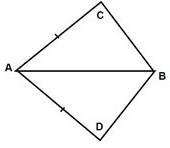## MCQ Questions for Class 7 Maths: Ch 7 Congruence of Triangles1. What comes next in the sequence: 2, 4, 10, 28, ___ ?
(a) 64
(b) 70
(c) 76
(d) 82
► (d) 82

2. Two angles are congruent if they have
(a) Same name
(b) unequal measures
(c) equal measures
(d) none of these
► (c) equal measures

3. What is the side included between the angles A and B in ΔABC?
(a) AC
(b) BC
(c) AB
(d) None of these
► (c) AB

4. ΔABC and ΔPQR are congruent under the correspondence: ABC ↔ RQP, then the part of ΔABC that correspond to ∠P is
(a) ∠A
(b) ∠C
(c) ∠B
(d) None of these
► (b) ∠C

5. If the vertical angle of a isosceles triangle is 40°, then measure of other two angles will be
(a) 60°, 60°
(b) 80°, 80°
(c) 70°, 70°
(d) 45°, 45°
► (c) 70°, 70°

6. Two triangles, A PQR and ADEF are of the same size and shape. What can we conclude about them?
(a) ΔPQR is smaller than ΔDFE.
(b) ΔPQR is larger than ΔDFE.
(c) ΔPQR is congruent to ΔDFE.
(d) ΔPQR is not congruent to ΔDFE.
► (c) ΔPQR is congruent to ΔDFE.

7. In ΔABC and ΔPQR, AB = 4 cm, BC = 5 cm, AC = 6 cm and PQ = 4 cm. QR = 5 cm. PR = 6 cm. then which of the following is true?
(a) ΔABC ≅ ΔQRP
(b) ΔABC ≅ ΔPQR
(c) ΔABC ≅ ΔRQP
(d) None of these
► (b) ΔABC ≅ ΔPQR

8. In ΔABC and ΔDEF, AC = DF,AB = DE and BC=EF. By which property are ΔABC and ΔDEF congruent?
(a) R.H.S. property
(b) S.S.S. property
(c) S.A.S. property
(d) A.S.A. property
► (b) S.S.S. property

9. A triangle in which all three sides are of equal lengths is called _________.
(a) Isosceles
(b) Equilateral
(c) Scalene
(d) None of these
► (b) Equilateral

10. Which angle is included between the sides DE and EF of △DEF?
(a) ∠F
(b) ∠D
(c) ∠E
(d) None of these
► (c) ∠E

11. In the quadrilateral ABCD, AC = AD and AB bisect ∠A and ΔABC ≅ ΔABD. The relation between BC and BD is(a) BC < BD
(b) BC > BD
(c) BC = BD
(d) None of these
► (c) BC = BD

12. If ΔDEF ≅ ΔBCA, then the part of ΔBCA that correspond to ∠E is
(a) ∠B
(b) ∠C
(c) ∠A
(d) None of these
► (b) ∠C

13. Two students drew a line segment each. What is the condition for them to be congruent?
(a) They should be drawn with a scale.
(b) They should be drawn on the same sheet of paper.
(c) They should have different lengths.
(d) They should have the same length.
► (d) They should have the same length.

14. The measure of each angle of an equilateral triangle is:
(a) 50°
(b) 70°
(c) 60°
(d) 100°
► (c) 60°

15. ΔABC is right triangle in which ∠A = 90° and AB = AC. The values of ∠B and ∠C will be
(a) ∠B = ∠C = 30°
(b) ∠B = ∠C = 50°
(c) ∠B = ∠C = 45°
(d) ∠B = ∠C = 60°
► (c)∠B = ∠C = 45°

16. Which of the following examines the congruence of plane figures?
(a) Trial and error method
(b) Superposition method
(c) Substitution method
(d) Transposition method
► (b) Superposition method

17. ΔABC and ΔPQR are congruent under the correspondence: ABC ↔ RPQ, then the part of ΔABC that correspond to PQ is
(a) AC
(b) AB
(c) BC
(d) None of These
► (c) BC

18. What is the angle included between the sides PN and PM of ΔMNP?
(a) ∠M
(b) ∠N
(c) ∠P
(d) None of these
► (c) ∠P